/
/
/
26. An object of mass the surface of the
Not my Question
Flag Content

# Question :   26. An object of mass the surface of the : 2102103

26. An object of mass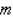is on the surface of the Earth. The distance to the Sun and the Moon are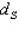and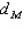, and the masses of the Sun and Moon are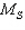and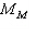. What is the ratio of the gravitational force from the Sun to that of the Moon on the object?

A.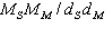B.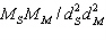C.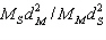D.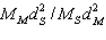27. Two blocks, joined by a string, have masses of 6.0 and 9.0 kg. They rest on a frictionless horizontal surface. A 2nd string, attached only to the 9-kg block, has horizontal force = 30 N applied to it. Both blocks accelerate. Find the tension in the string between the blocks.

A. 18 N

B. 28 N

C. 12 N

D. 15 N

28. Three forces, 5.0 N, 15.0 N, and 20.0 N, are acting on a 9.81-kg object. Which of the following forces could also be acting on the object if it is moving with constant velocity?

A. 1.0 N

B. 19.0 N

C. 39.0 N

D. any of the above

29. An airplane of mass 1.2 ´ 104 kg tows a glider of mass 0.6 ´ 104 kg. The airplane propellers provide a net forward thrust of 3.6 ´ 104 N. What is the glider's acceleration?

A. 2.0 m/s2

B. 3.0 m/s2

C. 6.0 m/s2

D. 9.8 m/s2

30. Two blocks of masses 20 kg and 8 kg are connected together by a light string and rest on a frictionless level surface. Attached to the 8-kg mass is another light string, which a person uses to pull both blocks horizontally. If the two-block system accelerates at 0.5 m/s2 what is the tension in the connecting string between the blocks?

A. 14 N

B. 6 N

C. 10 N

D. 4.0 N

## Solution 5 (1 Ratings )

Solved
Physics 1 Year Ago 89 Views